# RD Sharma Solutions for Class 8 Maths Chapter 18 Practical Geometry (Constructions) Exercise 18.1

In Exercise 18.1 of Chapter 18, we shall discuss how to construct a quadrilateral when four sides and one diagonal are given. Solutions are designed by our expert faculty team with the aim to help students score well in their exams. On regular practice, students improve logical thinking and problem-solving abilities, which is an important aspect of learning. For a better understanding of the concepts, students can refer to RD Sharma Solutions and can download the pdf easily from the links provided below.

## Download the Pdf of RD Sharma Solutions for Class 8 Maths Exercise 18.1 Chapter 18 Practical Geometry (Constructions)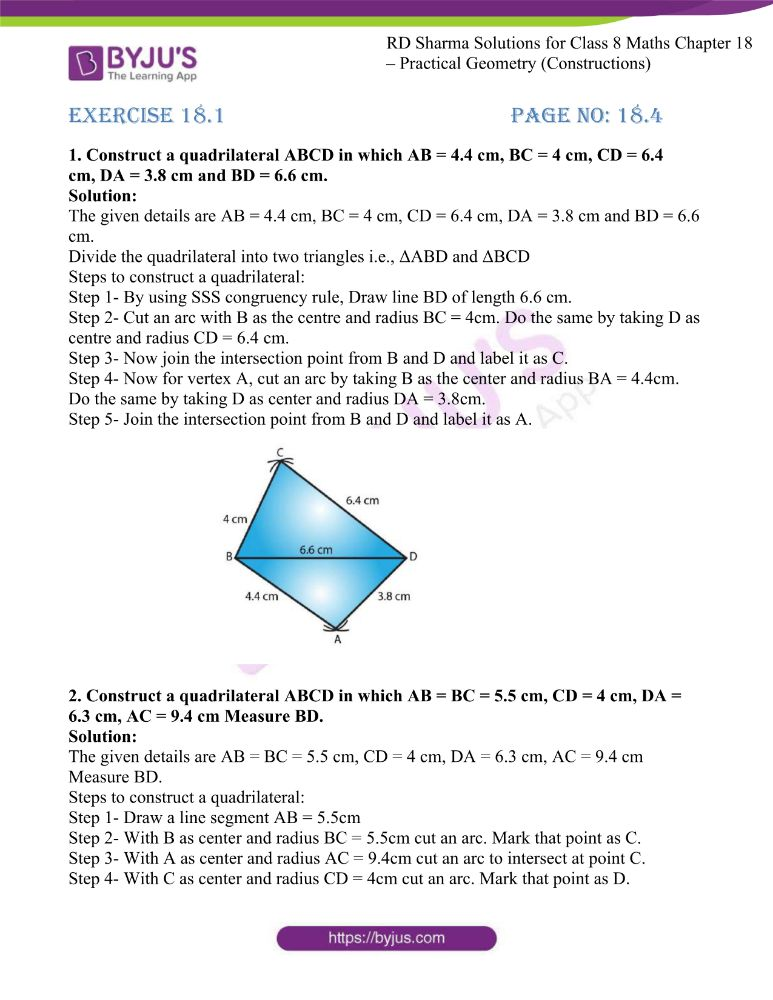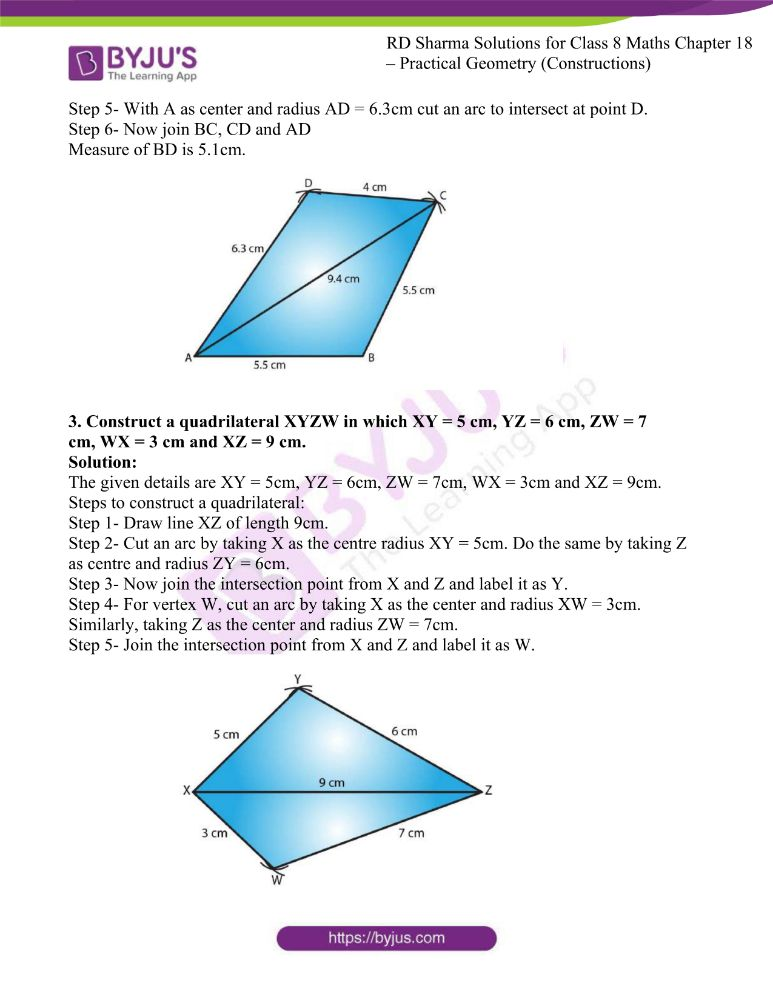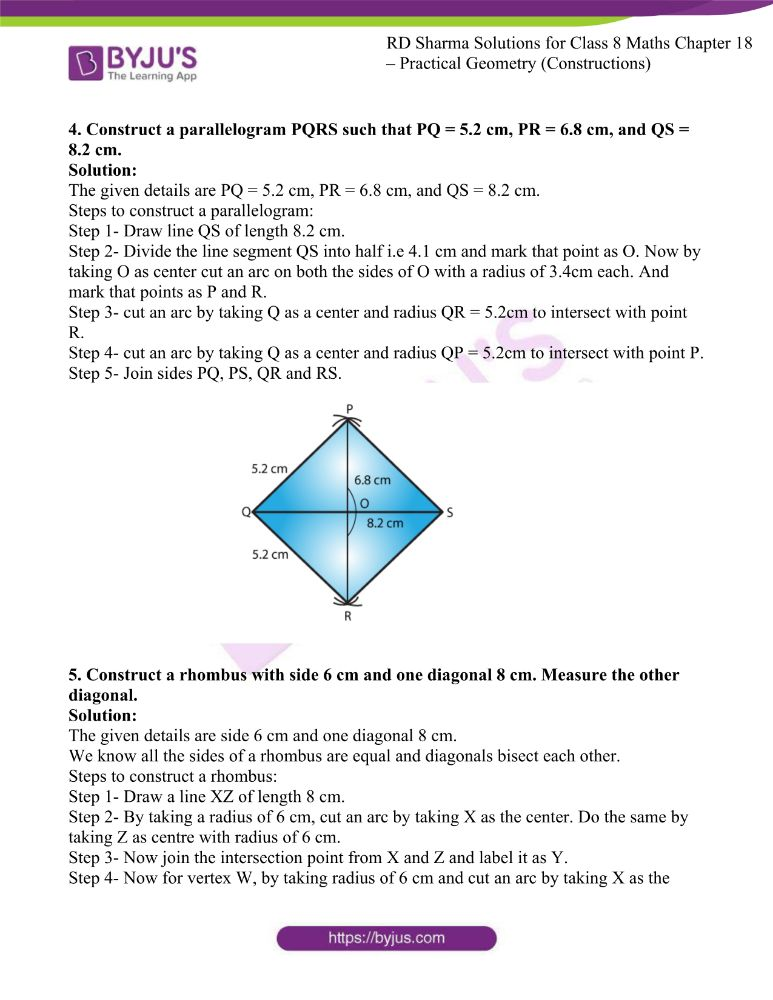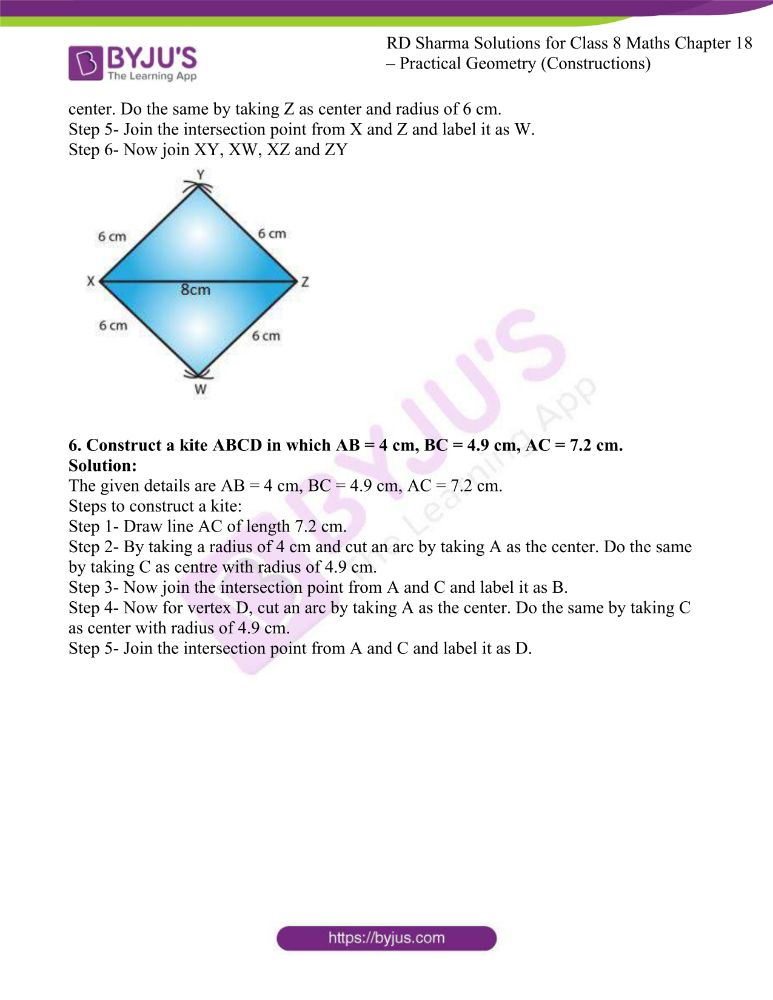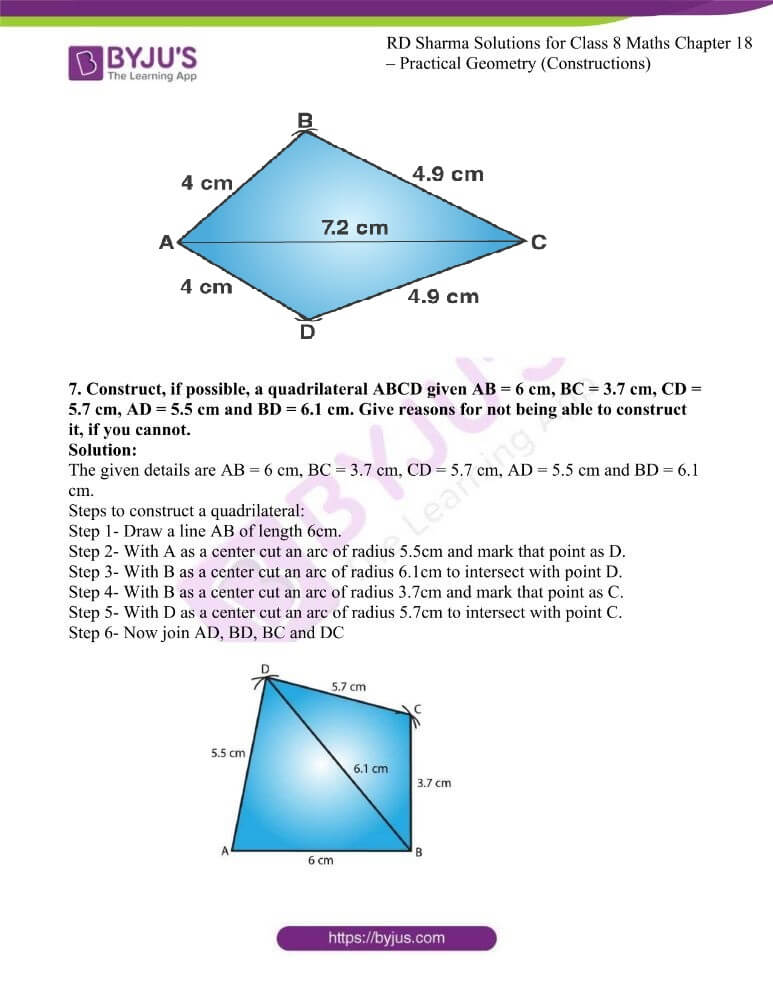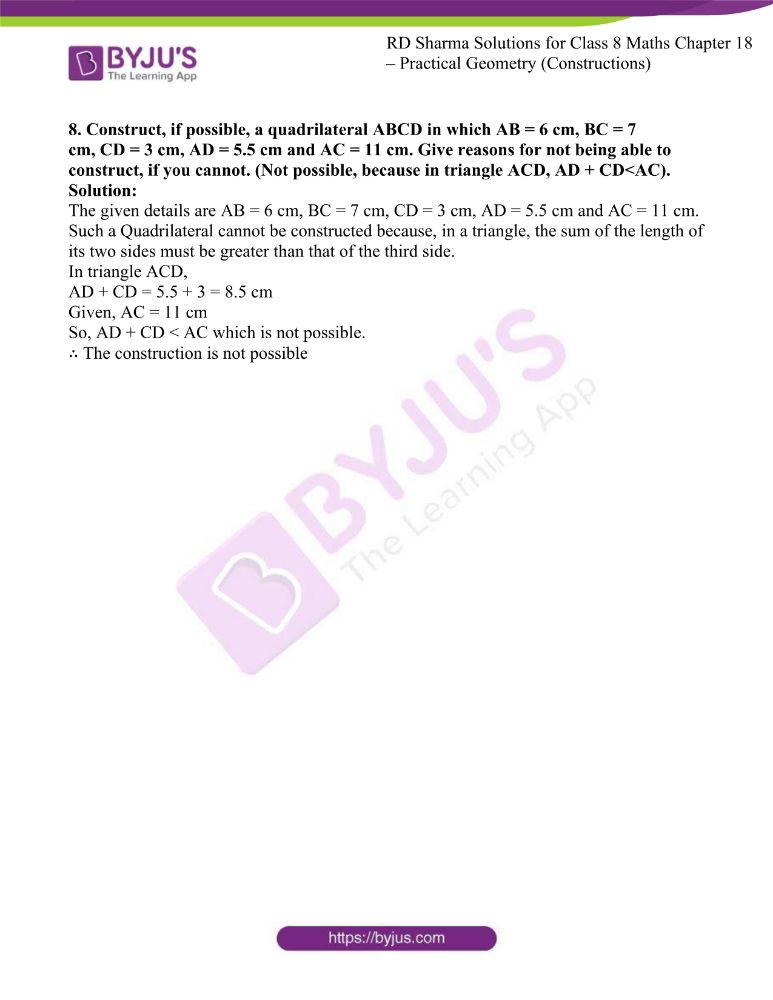### Access answers to RD Sharma Maths Solutions For Class 8 Exercise 18.1 Chapter 18 Practical Geometry (Constructions)

1. Construct a quadrilateral ABCD in which AB = 4.4 cm, BC = 4 cm, CD = 6.4 cm, DA = 3.8 cm and BD = 6.6 cm.

Solution:

The given details are AB = 4.4 cm, BC = 4 cm, CD = 6.4 cm, DA = 3.8 cm and BD = 6.6 cm.

Divide the quadrilateral into two triangles i.e., ΔABD and ΔBCD

Step 1- By using SSS congruency rule, Draw line BD of length 6.6 cm.

Step 2- Cut an arc with B as the centre and radius BC = 4cm. Do the same by taking D as centre and radius CD = 6.4 cm.

Step 3- Now join the intersection point from B and D and label it as C.

Step 4- Now for vertex A, cut an arc by taking B as the center and radius BA = 4.4cm. Do the same by taking D as center and radius DA = 3.8cm.

Step 5- Join the intersection point from B and D and label it as A.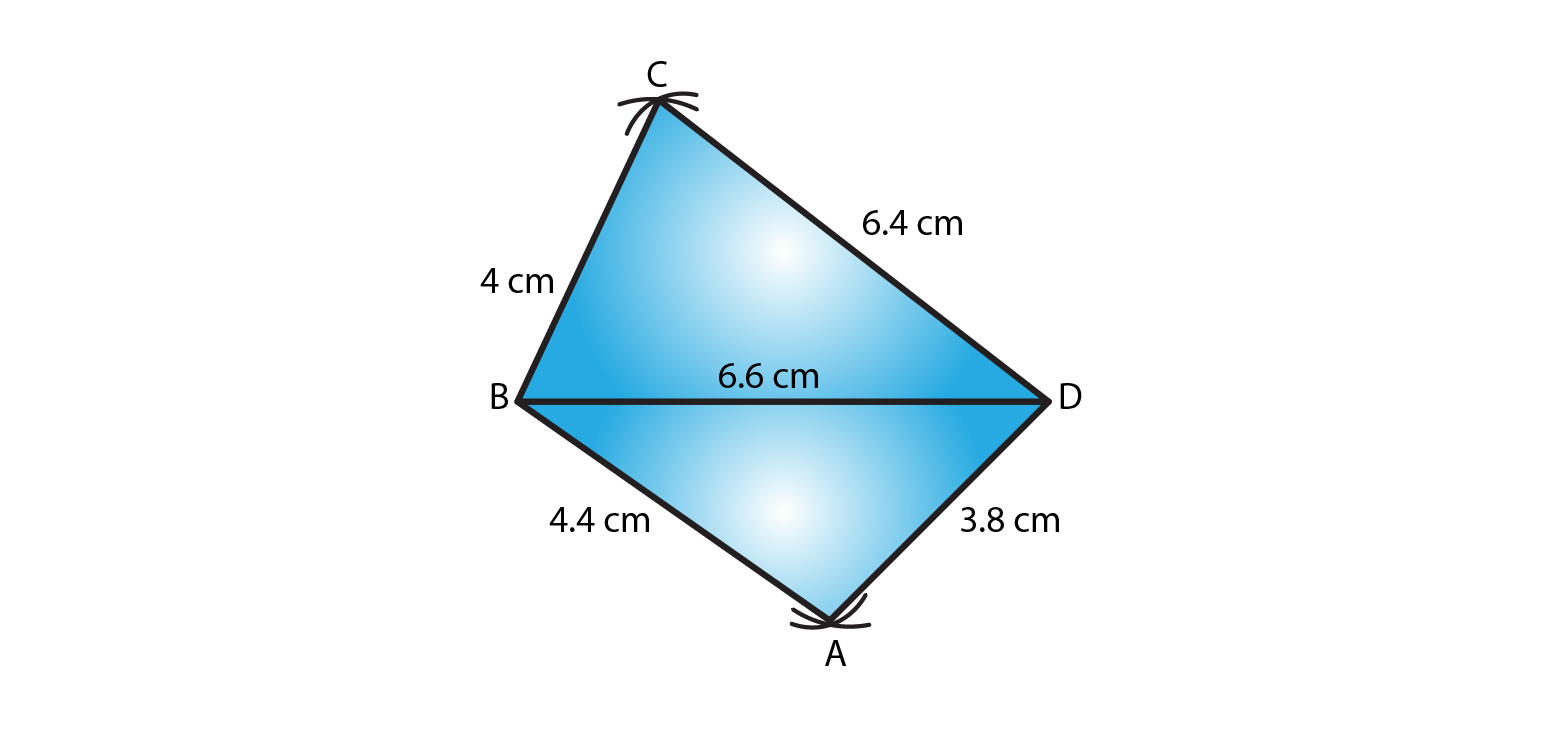2. Construct a quadrilateral ABCD in which AB = BC = 5.5 cm, CD = 4 cm, DA = 6.3 cm, AC = 9.4 cm Measure BD.

Solution:

The given details are AB = BC = 5.5 cm, CD = 4 cm, DA = 6.3 cm, AC = 9.4 cm Measure BD.

Step 1- Draw a line segment AB = 5.5cm

Step 2- With B as center and radius BC = 5.5cm cut an arc. Mark that point as C.

Step 3- With A as center and radius AC = 9.4cm cut an arc to intersect at point C.

Step 4- With C as center and radius CD = 4cm cut an arc. Mark that point as D.

Step 5- With A as center and radius AD = 6.3cm cut an arc to intersect at point D.

Step 6- Now join BC, CD and AD

Measure of BD is 5.1cm.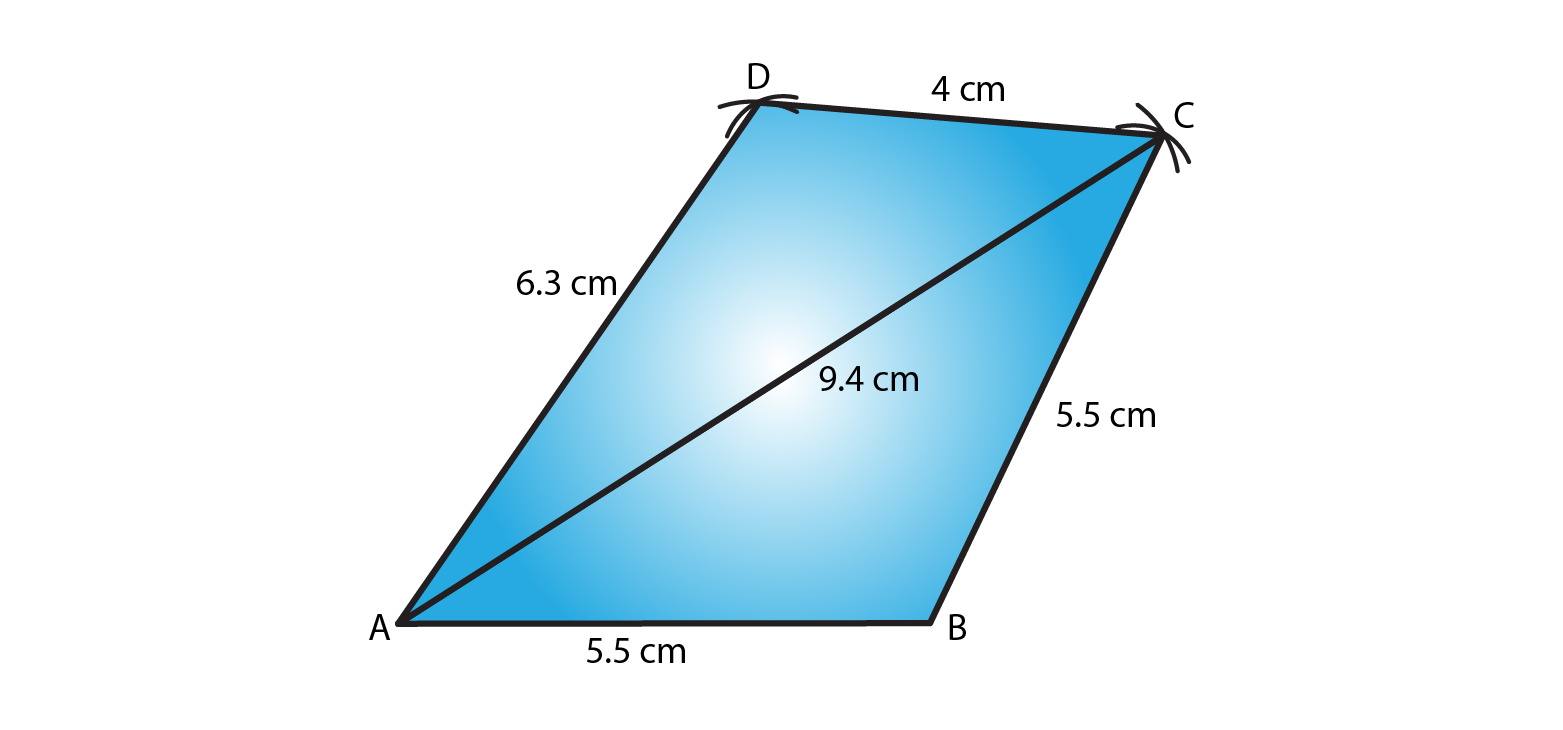3. Construct a quadrilateral XYZW in which XY = 5 cm, YZ = 6 cm, ZW = 7 cm, WX = 3 cm and XZ = 9 cm.

Solution:

The given details are XY = 5cm, YZ = 6cm, ZW = 7cm, WX = 3cm and XZ = 9cm.

Step 1- Draw line XZ of length 9cm.

Step 2- Cut an arc by taking X as the centre radius XY = 5cm. Do the same by taking Z as centre and radius ZY = 6cm.

Step 3- Now join the intersection point from X and Z and label it as Y.

Step 4- For vertex W, cut an arc by taking X as the center and radius XW = 3cm. Similarly, taking Z as the center and radius ZW = 7cm.

Step 5- Join the intersection point from X and Z and label it as W.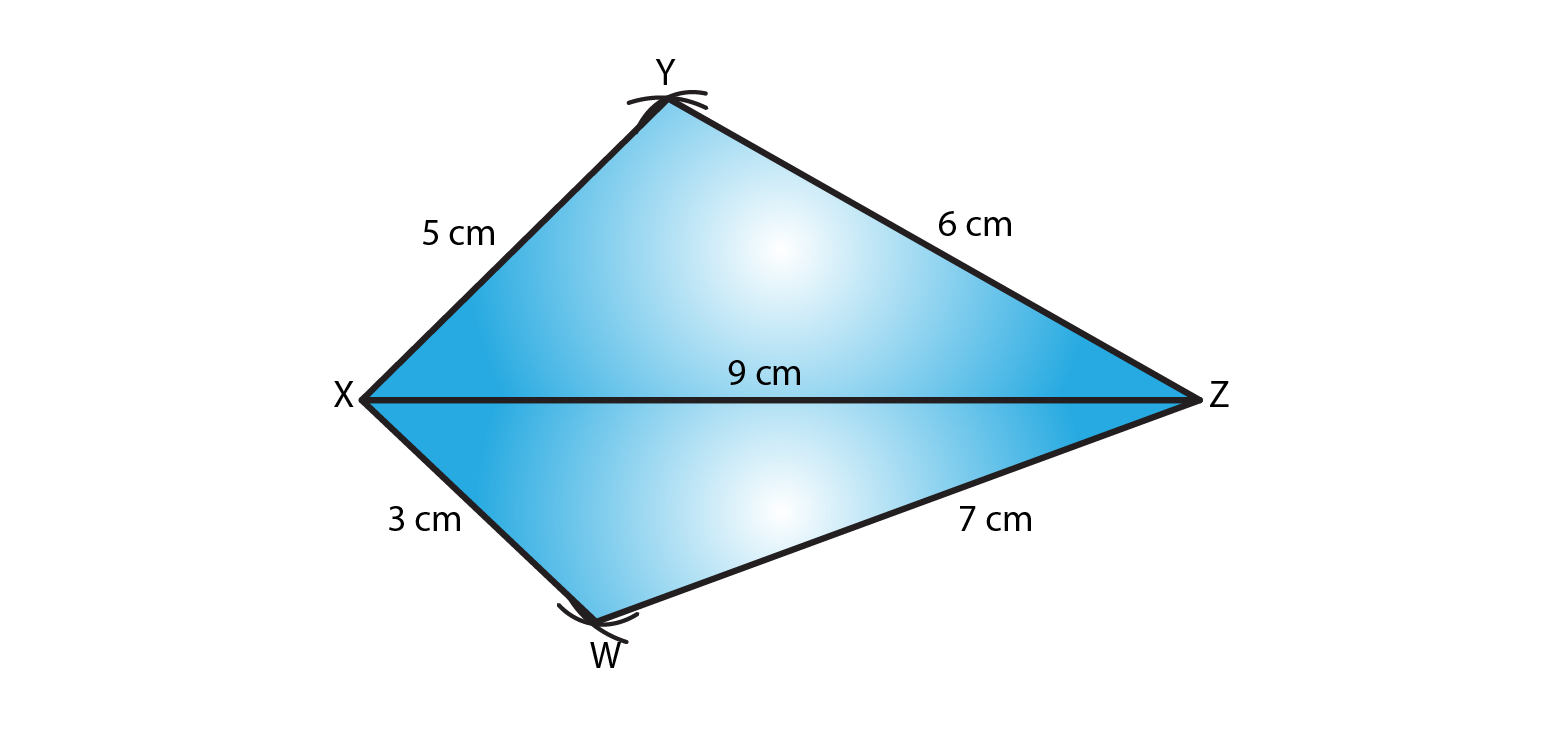4. Construct a parallelogram PQRS such that PQ = 5.2 cm, PR = 6.8 cm, and QS = 8.2 cm.

Solution:

The given details are PQ = 5.2 cm, PR = 6.8 cm, and QS = 8.2 cm.

Steps to construct a parallelogram:

Step 1- Draw line QS of length 8.2 cm.

Step 2- Divide the line segment QS into half i.e 4.1 cm and mark that point as O. Now by taking O as center cut an arc on both the sides of O with a radius of 3.4cm each. And mark that points as P and R.

Step 3- cut an arc by taking Q as a center and radius QR = 5.2cm to intersect with point R.

Step 4- cut an arc by taking Q as a center and radius QP = 5.2cm to intersect with point P.

Step 5- Join sides PQ, PS, QR and RS.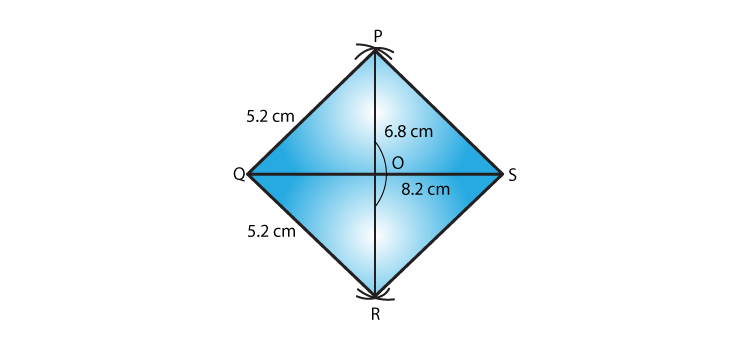5. Construct a rhombus with side 6 cm and one diagonal 8 cm. Measure the other diagonal.

Solution:

The given details are side 6 cm and one diagonal 8 cm.

We know all the sides of a rhombus are equal and diagonals bisect each other.

Steps to construct a rhombus:

Step 1- Draw a line XZ of length 8 cm.

Step 2- By taking a radius of 6 cm, cut an arc by taking X as the center. Do the same by taking Z as centre with radius of 6 cm.

Step 3- Now join the intersection point from X and Z and label it as Y.

Step 4- Now for vertex W, by taking radius of 6 cm and cut an arc by taking X as the center. Do the same by taking Z as center and radius of 6 cm.

Step 5- Join the intersection point from X and Z and label it as W.

Step 6- Now join XY, XW, XZ and ZY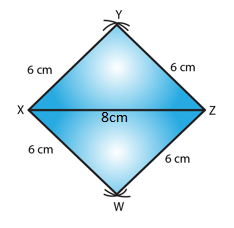6. Construct a kite ABCD in which AB = 4 cm, BC = 4.9 cm, AC = 7.2 cm.

Solution:

The given details are AB = 4 cm, BC = 4.9 cm, AC = 7.2 cm.

Steps to construct a kite:

Step 1- Draw line AC of length 7.2 cm.

Step 2- By taking a radius of 4 cm and cut an arc by taking A as the center. Do the same by taking C as centre with radius of 4.9 cm.

Step 3- Now join the intersection point from A and C and label it as B.

Step 4- Now for vertex D, cut an arc by taking A as the center. Do the same by taking C as center with radius of 4.9 cm.

Step 5- Join the intersection point from A and C and label it as D.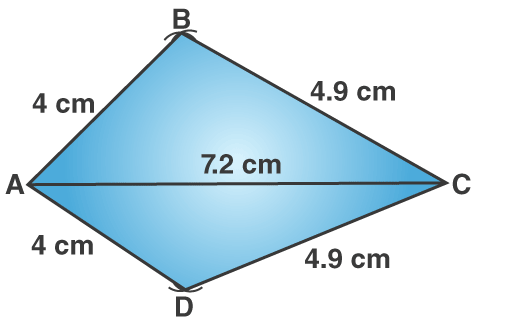7. Construct, if possible, a quadrilateral ABCD given AB = 6 cm, BC = 3.7 cm, CD = 5.7 cm, AD = 5.5 cm and BD = 6.1 cm. Give reasons for not being able to construct it, if you cannot.

Solution:

The given details are AB = 6 cm, BC = 3.7 cm, CD = 5.7 cm, AD = 5.5 cm and BD = 6.1 cm.

Step 1- Draw a line AB of length 6cm.

Step 2- With A as a center cut an arc of radius 5.5cm and mark that point as D.

Step 3- With B as a center cut an arc of radius 6.1cm to intersect with point D.

Step 4- With B as a center cut an arc of radius 3.7cm and mark that point as C.

Step 5- With D as a center cut an arc of radius 5.7cm to intersect with point C.

Step 6- Now join AD, BD, BC and DC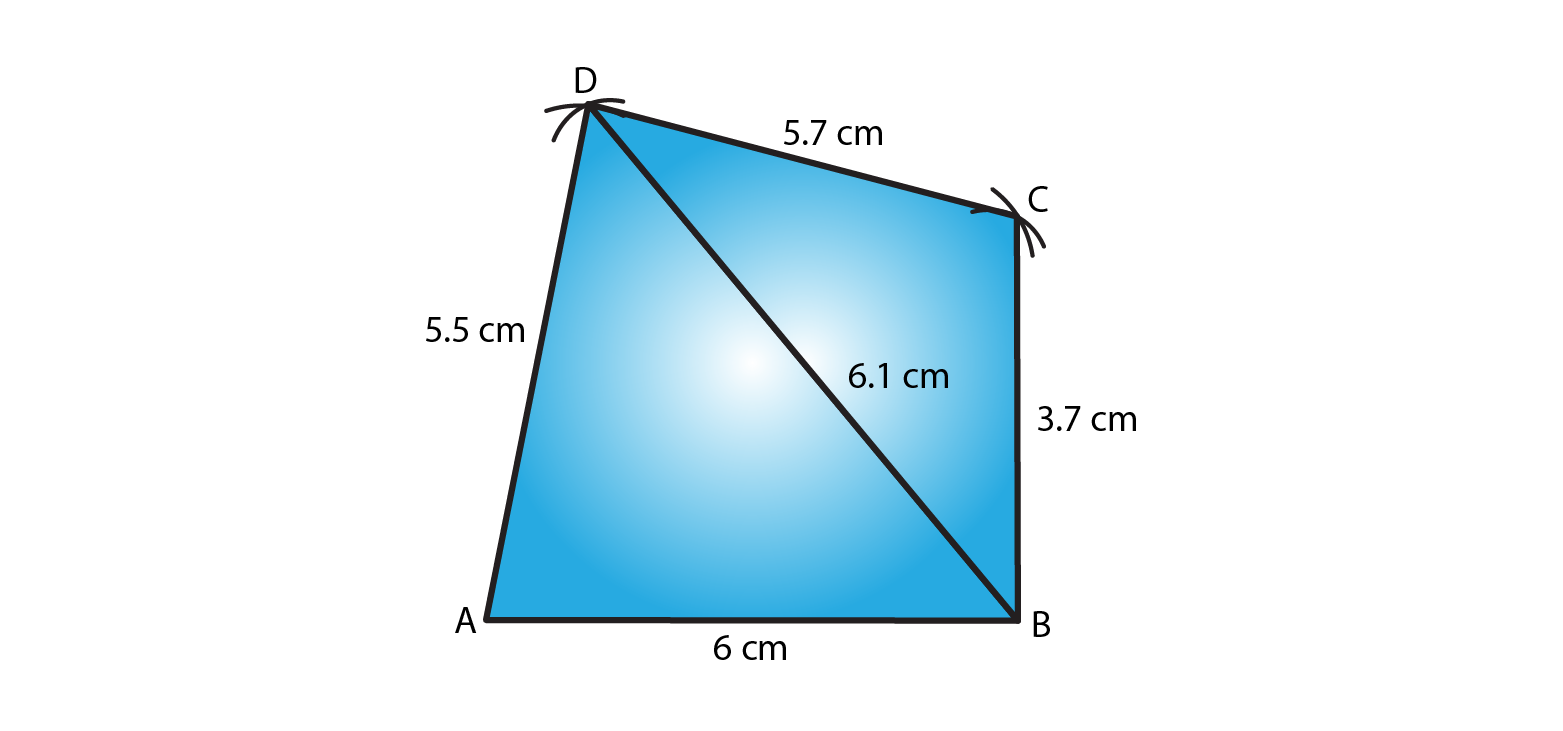8. Construct, if possible, a quadrilateral ABCD in which AB = 6 cm, BC = 7 cm, CD = 3 cm, AD = 5.5 cm and AC = 11 cm. Give reasons for not being able to construct, if you cannot. (Not possible, because in triangle ACD, AD + CD<AC).

Solution:

The given details are AB = 6 cm, BC = 7 cm, CD = 3 cm, AD = 5.5 cm and AC = 11 cm.

Such a Quadrilateral cannot be constructed because, in a triangle, the sum of the length of its two sides must be greater than that of the third side.

In triangle ACD,

AD + CD = 5.5 + 3 = 8.5 cm

Given, AC = 11 cm

So, AD + CD < AC which is not possible.

∴ The construction is not possible